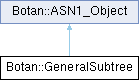Botan  2.9.0 Crypto and TLS for C++11
Botan::GeneralSubtree Class Referencefinal

A single Name Constraint. More...

`#include <name_constraint.h>`

Inheritance diagram for Botan::GeneralSubtree:## Public Member Functions

GeneralName base () const

std::vector< uint8_t > BER_encode () const

void decode_from (BER_Decoder &) override

void encode_into (DER_Encoder &) const override

GeneralSubtree ()

GeneralSubtree (GeneralName base, size_t min, size_t max)

GeneralSubtree (const std::string &str)

size_t maximum () const

size_t minimum () const

## Detailed Description

A single Name Constraint.

The Name Constraint extension adds a minimum and maximum path length to a GeneralName to form a constraint. The length limits are currently unused.

Definition at line 90 of file name_constraint.h.

## ◆ GeneralSubtree() [1/3]

 Botan::GeneralSubtree::GeneralSubtree ( )
inline

Creates an empty name constraint.

Definition at line 96 of file name_constraint.h.

96  : m_base(), m_minimum(0), m_maximum(std::numeric_limits<std::size_t>::max())
97  {}

## ◆ GeneralSubtree() [2/3]

 Botan::GeneralSubtree::GeneralSubtree ( GeneralName base, size_t min, size_t max )
inline

Definition at line 105 of file name_constraint.h.

106  : m_base(base), m_minimum(min), m_maximum(max)
107  {}
GeneralName base() const

## ◆ GeneralSubtree() [3/3]

 Botan::GeneralSubtree::GeneralSubtree ( const std::string & str )

Creates a new name constraint for its string format.

Parameters
 str name constraint

Definition at line 227 of file name_constraint.cpp.

227  : GeneralSubtree()
228  {
229  size_t p0, p1;
230  size_t min = std::stoull(str, &p0, 10);
231  size_t max = std::stoull(str.substr(p0 + 1), &p1, 10);
232  GeneralName gn(str.substr(p0 + p1 + 2));
233
234  if(p0 > 0 && p1 > 0)
235  {
236  m_minimum = min;
237  m_maximum = max;
238  m_base = gn;
239  }
240  else
241  {
242  throw Invalid_Argument("Failed to decode Name Constraint");
243  }
244  }

## ◆ base()

 GeneralName Botan::GeneralSubtree::base ( ) const
inline
Returns
name

Definition at line 122 of file name_constraint.h.

Referenced by Botan::operator<<().

122 { return m_base; }

## ◆ BER_encode()

 std::vector< uint8_t > Botan::ASN1_Object::BER_encode ( ) const
inherited

Return the encoding of this object. This is a convenience method when just one object needs to be serialized. Use DER_Encoder for complicated encodings.

Definition at line 16 of file asn1_obj.cpp.

References Botan::ASN1_Object::encode_into().

17  {
18  std::vector<uint8_t> output;
19  DER_Encoder der(output);
20  this->encode_into(der);
21  return output;
22  }
virtual void encode_into(DER_Encoder &to) const =0

## ◆ decode_from()

 void Botan::GeneralSubtree::decode_from ( BER_Decoder & from )
overridevirtual

Decode whatever this object is from from

Parameters
 from the BER_Decoder that will be read from

Implements Botan::ASN1_Object.

Definition at line 251 of file name_constraint.cpp.

252  {
253  ber.start_cons(SEQUENCE)
254  .decode(m_base)
255  .decode_optional(m_minimum,ASN1_Tag(0), CONTEXT_SPECIFIC,size_t(0))
256  .end_cons();
257
258  if(m_minimum != 0)
259  throw Decoding_Error("GeneralSubtree minimum must be 0");
260
261  m_maximum = std::numeric_limits<std::size_t>::max();
262  }
ASN1_Tag
Definition: asn1_obj.h:22

## ◆ encode_into()

 void Botan::GeneralSubtree::encode_into ( DER_Encoder & to ) const
overridevirtual

Encode whatever this object is into to

Parameters
 to the DER_Encoder that will be written to

Implements Botan::ASN1_Object.

Definition at line 246 of file name_constraint.cpp.

247  {
248  throw Not_Implemented("General Subtree encoding");
249  }

## ◆ maximum()

 size_t Botan::GeneralSubtree::maximum ( ) const
inline
Returns
maximum path length

Definition at line 132 of file name_constraint.h.

Referenced by Botan::operator<<().

132 { return m_maximum; }

## ◆ minimum()

 size_t Botan::GeneralSubtree::minimum ( ) const
inline
Returns
minimum path length

Definition at line 127 of file name_constraint.h.

Referenced by Botan::operator<<().

127 { return m_minimum; }

The documentation for this class was generated from the following files: